Question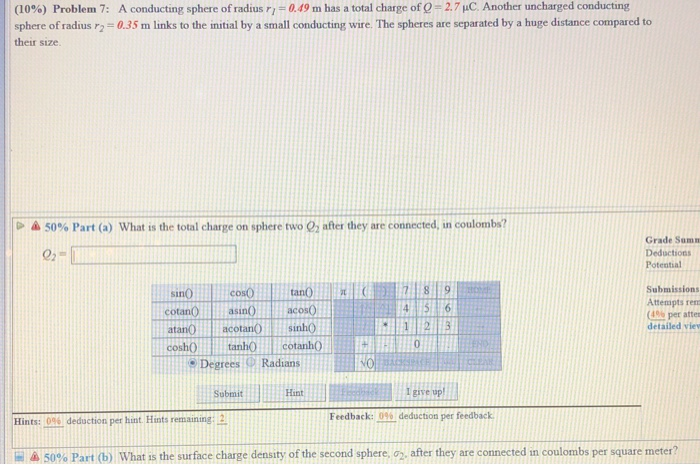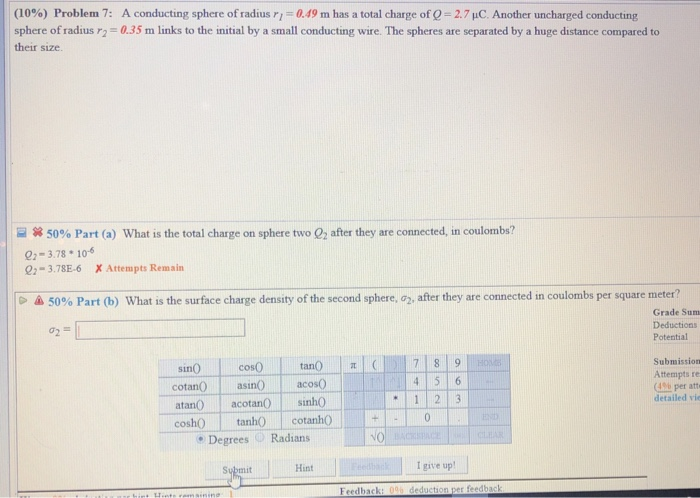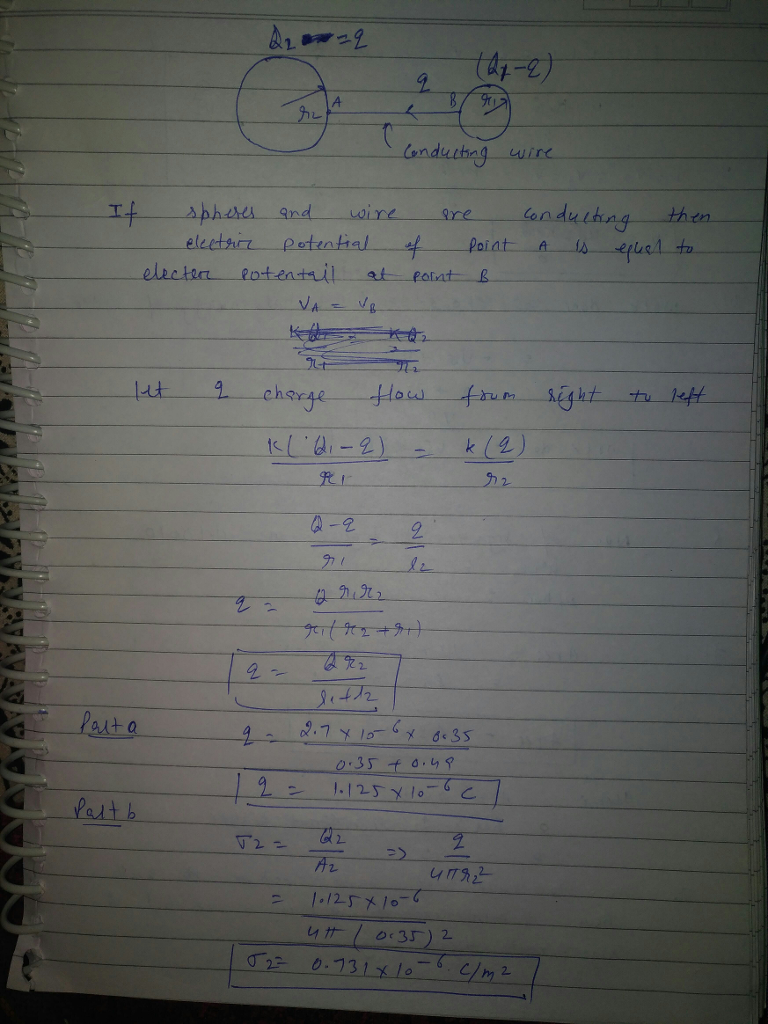#### Earn Coins

Coins can be redeemed for fabulous gifts.

Similar Homework Help Questions
• ### (10%) Problem 7: sphere of radius r2 their size. A conducting sphere of radius r,-0.49 m...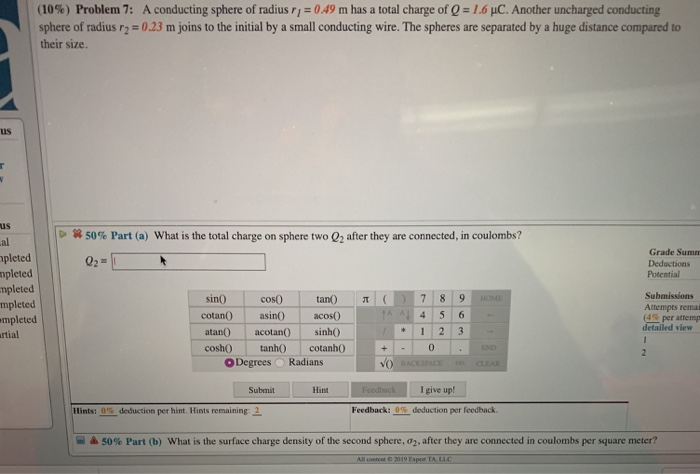(10%) Problem 7: sphere of radius r2 their size. A conducting sphere of radius r,-0.49 m has a total charge of Q-1.6 pC Another uncharged conducting 0.23 m joins to the initial by a small conducting wire. The spheres are separated by a huge distance compared to us IS al pleted mpleted mpleted mpleted mpleted rtial 50% Part (a) what is the total charge on sphere two Q2 after they are connected, in coulombs? Grade Sumn Deductions Potential Submissions Attempts...

• ### Problem 7: A conducting sphere of radius r,-0.49 m has a total charge of 0 1.6...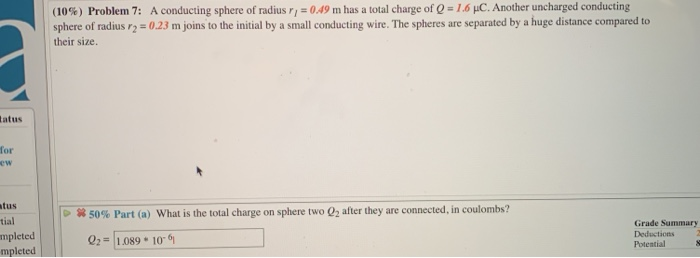Problem 7: A conducting sphere of radius r,-0.49 m has a total charge of 0 1.6 μ.c. Another uncharged conducting (10%) sphere of radius r2 023 m joins to the initial by a small conducting wire. The spheres are separated by a huge distance compared to their size. atus for ew tus tial mpleted 50% Part (a) what is the total charge on sphere two O2 after they are connected. in coulombs? Grade Summary Deductions Potential s Q2=11.089 * 10-61

• ### (10%) Problem 7: A conducting sphere of radius r,-049 m has a total charge of Q-1.6...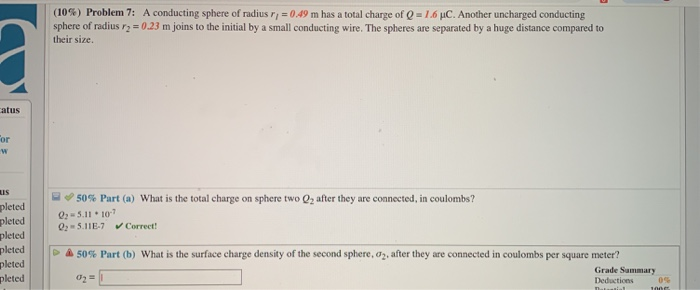(10%) Problem 7: A conducting sphere of radius r,-049 m has a total charge of Q-1.6 μ。 Another uncharged conducting sphere of radius 2-0.23 m joins to the initial by a small conducting wire. The spheres are separated by a huge distance compared to their size. atus or uS pleted pleted pleted -O 50% Part (a) what is the total charge on sphere two Q2 after they are connected, in coulombs? 0-5.11 10 5.11E-7 Correct pleted ム50% Part(b) what is...

• ### Part A and B on both please. Will upvote. A conducting sphere of radius rı 0.22...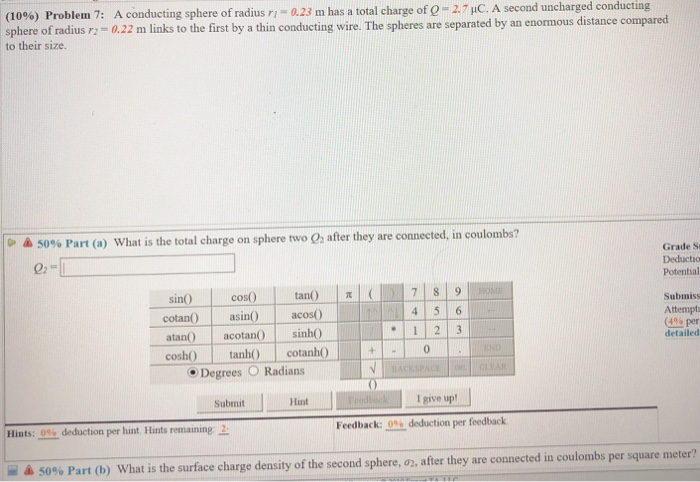Part A and B on both please. Will upvote. A conducting sphere of radius rı 0.22 m Problem 7: a23 m has a total charge of e-2-pC. A second uncharged conducting (10%) sphere of radius to their size. links to the first by a thin conducting wire. The spheres are separated by an enormous distance compared 50% Part (a) what is the total charge on sphere two 02 after they are connected, in coulom Grade S Deductio Potential sin() |...

• ### Can someone please help me with part A and B. Thank you (10%) Problem 7: A...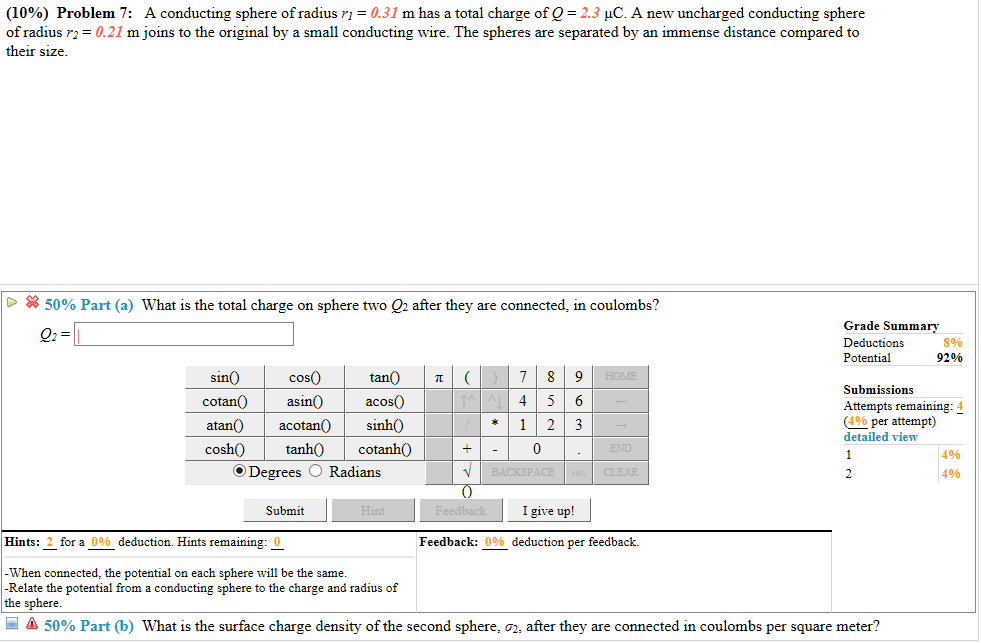Can someone please help me with part A and B. Thank you (10%) Problem 7: A conducting sphere of radius r! = 0.31 m has a total charge of Q = 2.3 μC. A new uncharged conducting sphere of radius r2 0.21 m joins to the original by a small conducting wire. The spheres are separated by an immense distance compared to their size - 50% Part (a) what is the total charge on sphere two Q2 after they are...

• ### R Q1-Ch23 A conducting solid sphere of radius R with unknown charge Q is at the...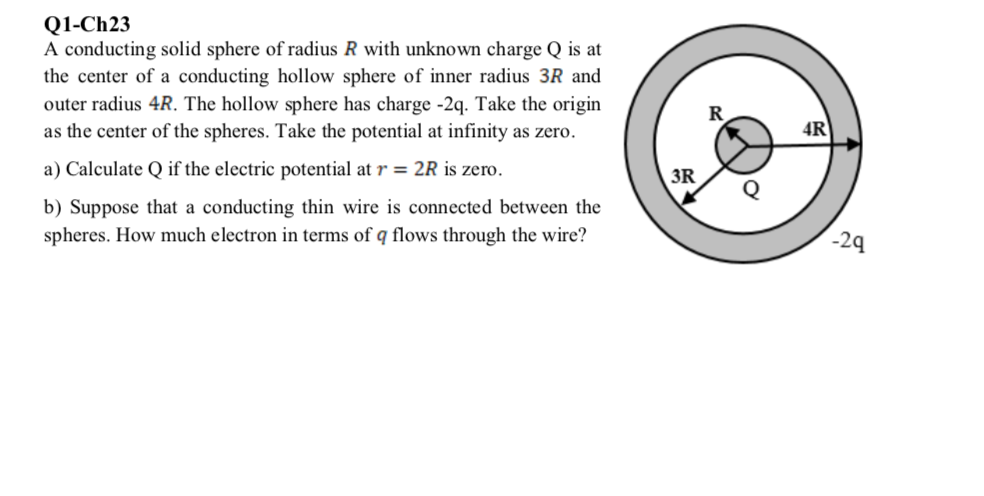R Q1-Ch23 A conducting solid sphere of radius R with unknown charge Q is at the center of a conducting hollow sphere of inner radius 3R and outer radius 4R. The hollow sphere has charge -2q. Take the origin as the center of the spheres. Take the potential at infinity as zero. a) Calculate Q if the electric potential at r = 2R is zero. b) Suppose that a conducting thin wire is connected between the spheres. How much electron...

• ### (9%) Problem 5: A hollow conducting sphere has an inside radius of a net charge of...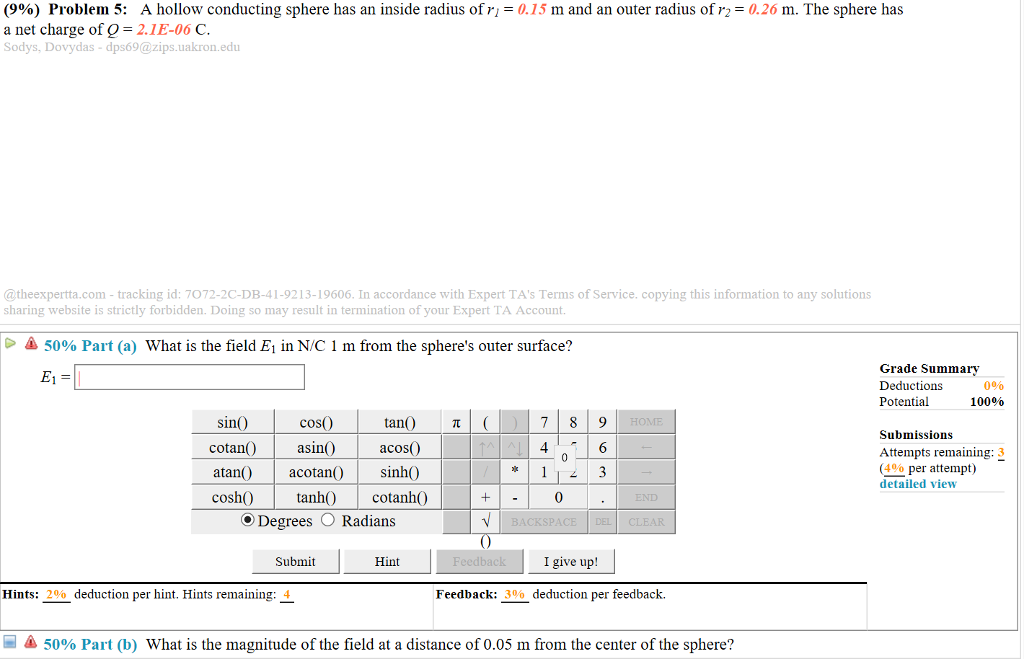(9%) Problem 5: A hollow conducting sphere has an inside radius of a net charge of Q = 2.1E-06 C Sodys, Dovydas-dps69@zips.uakron.edu = 0.15 m and an outer radius of r2 = 0.26 m. The sphere has @theexpertta.com-tracking id: 7072-2C-DB-41-9213-19606. In accordance with Expert TA's Terms of Service. copying this information to any solutions sharing website is strictly forbidden. Doing so may result in termination of your Expert TA Account 50% Part (a) what is the field E, in N/C...

• ### Sphere 1 with radius R1 has positive charge q. Sphere 2 with radius

Sphere 1 with radius R1 has positive charge q. Sphere 2 with radius 7.0R1 is far from sphere 1 and initially uncharged. After the separated spheres are connected witha wire thin enough to retain only negligible charge, what fraction of q ends up on (a) sphere 1 and (b) sphere 2? (c) What is the ratio of the final surface chargedensity of sphere 1 to that of sphere 2? (Answer with 2 significant figures.)

• ### 4. Two conducting spheres are far apart. The smaller sphere has radius R and carries a...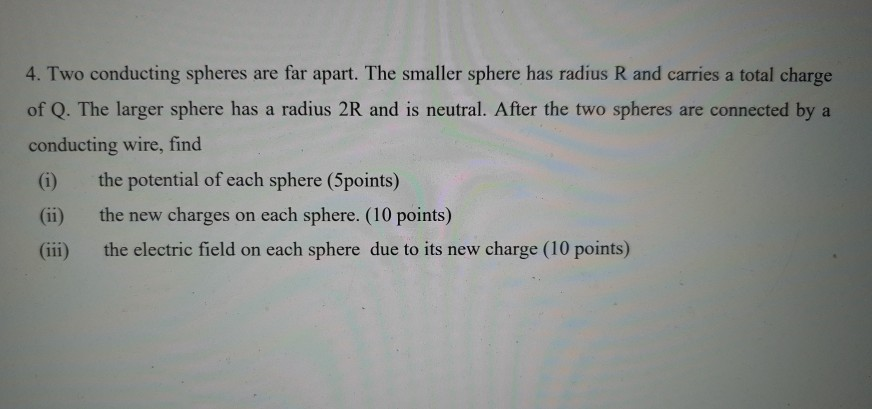4. Two conducting spheres are far apart. The smaller sphere has radius R and carries a total charge of Q. The larger sphere has a radius 2R and is neutral. After the two spheres are connected by a conducting wire, find the potential of each sphere (5points) the new charges on each sphere. (10 points) (iii) the electric field on each sphere due to its new charge (10 points)

• ### 4. Two conducting spheres are far apart. The smaller sphere has radius R and carries a...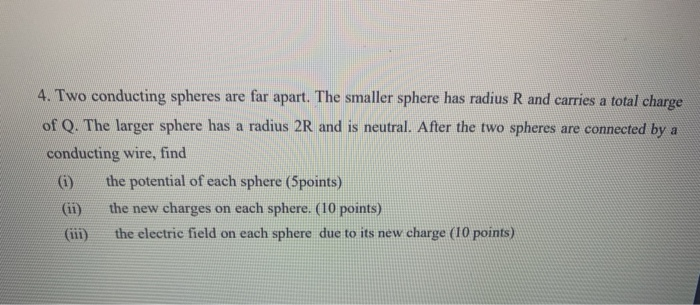4. Two conducting spheres are far apart. The smaller sphere has radius R and carries a total charge of Q. The larger sphere has a radius 2R and is neutral. After the two spheres are connected by a conducting wire, find (1) the potential of each sphere (5points) the new charges on each sphere. (10 points) the electric field on each sphere due to its new charge (10 points)